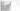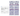# EFit

Interactive Event Fitting
Interactively determine velocity and thickness of a layered model by fitting T-X curves with refraction and reflection eventsData input
• Load single or multiple records
• Check and assign geometry
• Optionally load velocity model for reprocessing
Data processing
• AGC, trace balance, and time-variant scaling
• Frequency filtering
• Trigger delay correction
• Undo or redo multiple steps in data processing
Event fitting
• Define initial velocity model
• Calculate T-X curves of reflected and refracted waves
• Plot T-X curves on seismic records
• Interactively adjust velocity model to fit T-X curves with reflection and refraction events
• Output velocity model